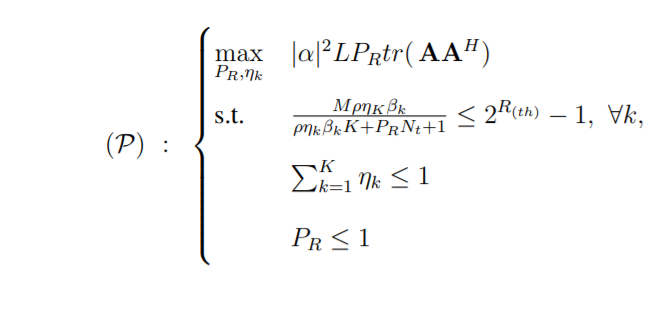clear all
clc
D=1/2;
N=3;
M=3;
Beta=1;
rho=10;
theta_deg=;
snr_dB=30; %in dB
rho = (10.^(snr_dB/10));
theta=theta_deg*pi/180;
K=3;
A=zeros(N,N);
for kk=1:N

end
P=1;
cvx_begin
variable P_R
variable eta

maximize((P_R*real((trace(A.*A'))))/sum(eta))

subject to

% P_R<= 1
P_R>=0

(M*Beta*eta_1*rho)/(rho*K*sum(eta)+P_R*N+1)>=1         %%%% R_k1>=1

sum(eta)<=P

cvx_end

CVX does not allow division of variables (except in limited circumstances in gp mode).

Presuming eta is constrained to >= 0, you can move the denominator in the constraint to the right hand side. Then the objective can be dealt with by linear fractional programming, per section 4.3.2 “Linear-fractional programming” in https://web.stanford.edu/~boyd/cvxbook/bv_cvxbook.pdf

For future difficulties, you should show us the exact error messages you received.

clear all
clc
D=1/2;
N=3;
M=3;
Beta=1;
rho=10;
theta_deg=;
snr_dB=30; %in dB
rho = (10.^(snr_dB/10));
theta=theta_deg*pi/180;
K=3;
A=zeros(N,N);
for kk=1:N

end
P=1;
cvx_begin
variables P_R
variables eta_1
variables eta_2
variables eta_3
variables t
% maximize (P_Rreal((trace(AA’)))inv_pos(eta_1+eta_2+eta_3))
maximize((P_R
real((trace(A*A’))))/(eta_1+eta_2+eta_3))

subject to

P_R>=0
eta_1+eta_2+eta_3>=t

%
(MBetaeta_1rho)>=(rhoKeta_1+P_RN+1)%%%% R_k1>=1
(MBetaeta_2rho)>=(rhoKeta_2+P_RN+1)%%%% R_k2>=1
(MBetaeta_3rho)>=(rhoKeta_3+P_RN+1)%%%% R_k3>=1

eta_1+eta_2+eta_3<=P

cvx_end

The Error is that:
Error in / (line 15)
z = mtimes( x, y, ‘rdivide’ );

Error in op1 (line 27)
maximize((P_Rreal((trace(AA’))))/(eta_1+eta_2+eta_3))

thanks for your help, if i understand what you say, i did it like this but i still have error

You need to use the formulation for linear-feactional programming per the link in my first post.

Thanks, I’m working with this, really I appreciate you help

Hi Mark
I made some changes based on what you recommend to read, I obtained results but I am not sure if my way is correct or notclear all
clc
D=1/2;
N=100;
M=8;
Beta=1;
rho=30;
theta_deg=;
snr_dB=30; %in dB
rho = (10.^(snr_dB/10));
theta=theta_deg*pi/180;
K=30;
A=zeros(N,N);
for kk=1:N

end
P=1;
cvx_begin
variable P_R
variable eta

maximize(P_R*real((trace(A*A'))))

subject to

(M*Beta*eta*rho)>=(rho*K*eta+P_R*N+1)%%%% R_k1>=1
P_R<=1
sum(eta)<=1

cvx_end

These comments are relative to problem P as shown in your most recent post. My comments don’t address whether problem P corresponds to whatever problem you really want to solve.

What is the value of K? Your code is written as though it is 1; although you have sum(eta).

Your first constraint has >= instead of <=, and does not include the factor (2^R - 1) on the right-hand side. You also omitted Beta, which you can get away with using this input data, because Beta = , but if it ever changed, your code would be wrong. If eta is declared as variable eta(K), that would make it a column vector, and if Beta were K by 1, then you could use .* in the constraint, which would produce the required ‘K’ constraints using just one MATLAB statement, which is faster for CVX and saves code compared to using a for loop.

Should eta and P_R be constrained to be >= 0 ? if not, how do you guarantee the original denominator is positive, as is required to move it to the right-hand side?

You also have sloppy coding practices, such as setting the value of rho, then setting it again before it is used.

I am not saying this is the entirety of what’s wrong with your code.